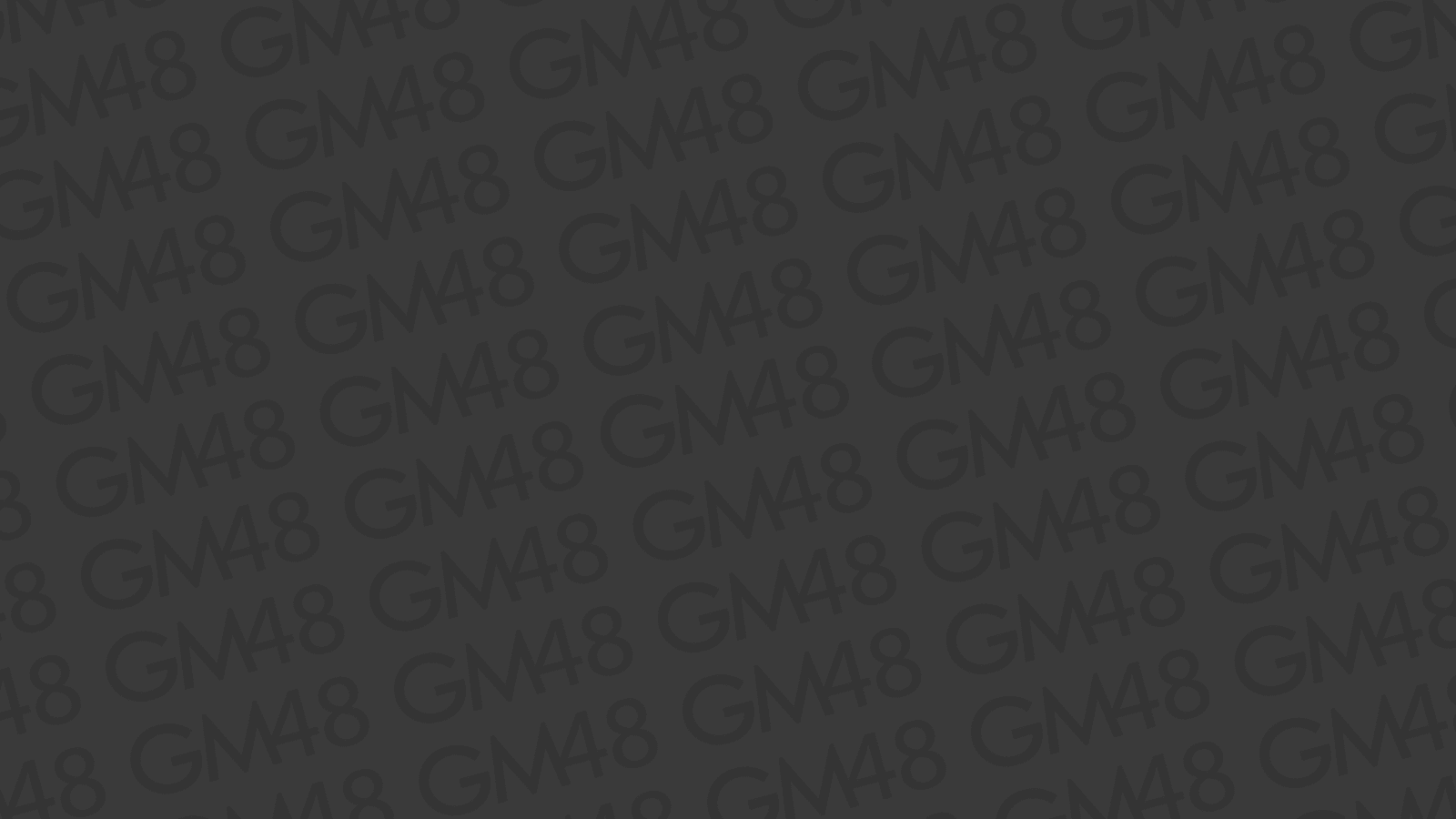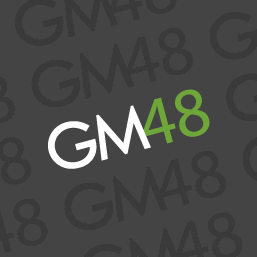example

# The Most Complicated Camera Code

Pretty self explanatory.###### Yosi
· 7 min read · 1473 views

## Introduction

It's not very fun to have to build the same camera code over and over each time you make a new project. So I decided to make some camera code that does most things a project would need. It's basically a modified version of Pixelated Pope's simple camera tutorial. It supports smooth camera movement, resizing, rotating, offsets, and screen shake.

## Setup

1. Make sure you have the `approach` script:
``````///@func approach
///@args current,goal,amount
return argument0 - clamp(argument0 - argument1, -argument2, argument2);``````
2. Make a new object that will control the camera, and paste in all of the following code in the correct events.

## The Code

• In the Create Event:
``````enum CAM_TYPE {
linear,
smooth,
}
cam = view_camera;
cam_min_x = 0;
cam_min_y = 0;
cam_max_x = room_width;
cam_max_y = room_height;
cam_x = 0;
cam_y = 0;
cam_goal_x = 0;
cam_goal_y = 0;
cam_move_speed = .1;
cam_move_type = CAM_TYPE.smooth;
cam_offset_x = 0;
cam_offset_y = 0;
cam_goal_offset_x = 0;
cam_goal_offset_y = 0;
cam_offset_speed = .5;
cam_offset_type = CAM_TYPE.smooth;
cam_offset_uses_rotation = true;
cam_w = 320;
cam_h = 240;
cam_goal_w = 320;
cam_goal_h = 240;
cam_resize_speed = .1;
cam_resize_type = CAM_TYPE.smooth;
cam_angle = 0;
cam_goal_angle = 0;
cam_rotate_speed = .1;
cam_rotate_type = CAM_TYPE.smooth;
cam_shake_x = 0;
cam_shake_y = 0;
cam_shake_range = false;
cam_shake_ignore_limits = true;
cam_stabilize_speed = 0.1;
cam_stabilize_type = CAM_TYPE.smooth;
cam_round_position = true;
var _window_scale = 2;
window_set_size(cam_w * _window_scale, cam_h * _window_scale);
alarm = 1;
surface_resize(application_surface, cam_w * _window_scale, cam_h * _window_scale);``````
• In the End Step event:
``````//Resizing
if (cam_w != cam_goal_w || cam_h != cam_goal_h) {
if (cam_resize_type == CAM_TYPE.linear) {
var _ratio = (cam_goal_h / cam_goal_w);
cam_w = approach(cam_w, cam_goal_w, cam_resize_speed);
cam_h = approach(cam_h, cam_goal_h, cam_resize_speed * _ratio);
} else if (cam_resize_type == CAM_TYPE.smooth) {
cam_w = lerp(cam_w, cam_goal_w, cam_resize_speed);
cam_h = lerp(cam_h, cam_goal_h, cam_resize_speed);
}
}
//Update Size
camera_set_view_size(cam, cam_w, cam_h);
//Rotating
if (cam_angle != cam_goal_angle) {
if (cam_rotate_type == CAM_TYPE.linear) {
cam_angle -= clamp(angle_difference(cam_angle, cam_goal_angle), -cam_rotate_speed, cam_rotate_speed);
} else if (cam_rotate_type == CAM_TYPE.smooth) {
cam_angle -= (angle_difference(cam_angle, cam_goal_angle) * cam_rotate_speed);
}
}
//Update Angle
camera_set_view_angle(cam, cam_angle);
//Movement
if (cam_x != cam_goal_x || cam_y != cam_goal_y) {
if (cam_move_type == CAM_TYPE.linear) {
cam_x = approach(cam_x, cam_goal_x, cam_move_speed);
cam_y = approach(cam_y, cam_goal_y, cam_move_speed);
} else if (cam_move_type == CAM_TYPE.smooth) {
cam_x = lerp(cam_x, cam_goal_x, cam_move_speed);
cam_y = lerp(cam_y, cam_goal_y, cam_move_speed);
}
}
//Offset
if (cam_offset_x != cam_goal_offset_x || cam_offset_y != cam_goal_offset_y) {
if (cam_offset_type == CAM_TYPE.linear) {
cam_offset_x = approach(cam_offset_x, cam_goal_offset_x, cam_offset_speed);
cam_offset_y = approach(cam_offset_y, cam_goal_offset_y, cam_offset_speed);
} else if (cam_offset_type == CAM_TYPE.smooth) {
cam_offset_x = lerp(cam_offset_x, cam_goal_offset_x, cam_offset_speed);
cam_offset_y = lerp(cam_offset_y, cam_goal_offset_y, cam_offset_speed);
}
}
//Final Calculations
var _off_x = cam_offset_x;
var _off_y = cam_offset_y;
if (cam_offset_uses_rotation) {
var _len = point_distance(0, 0, _off_x, _off_y);
var _dir = point_direction(0, 0, _off_x, _off_y);
_off_x = lengthdir_x(_len, _dir - cam_angle);
_off_y = lengthdir_y(_len, _dir - cam_angle);
}
var _final_x = cam_x - (cam_w / 2) + _off_x;
var _final_y = cam_y - (cam_h / 2) + _off_y;
_final_x = clamp(_final_x, cam_min_x, cam_max_x - cam_w);
_final_y = clamp(_final_y, cam_min_y, cam_max_y - cam_h);
//Camera Shake
if (cam_shake_range) {
_final_x += irandom_range(-cam_shake_x, cam_shake_x);
_final_y += irandom_range(-cam_shake_y, cam_shake_y);
} else {
_final_x += choose(-cam_shake_x, cam_shake_x);
_final_y += choose(-cam_shake_y, cam_shake_y);
}
if (!cam_shake_ignore_limits) {
_final_x = clamp(_final_x, cam_min_x, cam_max_x - cam_w);
_final_y = clamp(_final_y, cam_min_y, cam_max_y - cam_h);
}
if (cam_shake_x != 0 || cam_shake_y != 0) {
if (cam_stabilize_type == CAM_TYPE.linear) {
cam_shake_x = approach(cam_shake_x, 0, cam_stabilize_speed);
cam_shake_y = approach(cam_shake_y, 0, cam_stabilize_speed);
} else if (cam_stabilize_type == CAM_TYPE.smooth) {
cam_shake_x = lerp(cam_shake_x, 0, cam_stabilize_speed);
cam_shake_y = lerp(cam_shake_y, 0, cam_stabilize_speed);
}
}
//Update Position
if (cam_round_position) {
_final_x = round(_final_x);
_final_y = round(_final_y);
}
camera_set_view_pos(cam, _final_x, _final_y);``````
• In the Alarm 0 event:
``window_center();``
• In the Room Start event:
``````view_enabled = true;
view_visible = true;``````

## How to Use

Goal variables

• Set the `goal` variables to the camera properties you want the camera to end up with eventually.
• The `speed` variables determine how fast the camera properties approach the goal values.
• The `type` variable determines if the camera approaches the goal linearly or in percentages.

Moving the camera around

• Set `cam_goal_x` and `cam_goal_y` to where you want the camera to look at.
• Set `cam_round_position` if you want the camera position to be pixel perfect.
• You can set the camera borders with `cam_min_x`, `cam_min_y`, `cam_max_x`, and `cam_max_y`. These are the Top Left and Bottom Right coordinates. The example code has the camera restricted to the room borders.

Camera offset

• The camera offset is added to the camera coordinates during calculations.
• Set `cam_offset_x` and `cam_offset_y` to the desired offset.
• Setting `cam_offset_uses_rotation` to `true` will cause the camera offset to automatically change with the camera angle.

Camera size and window size

• Set `cam_goal_w` and `cam_goal_h` to the size you want the camera to be.
• Try to avoid changing the ratio, as that will mess up how the camera resizes.
• Change the value of `_window_scale` in the Create event to change the size of the window in relation to the `cam_w` and `cam_h`.

Rotation

• Set `cam_goal_angle` to the angle you want the view to rotate to.
• If you want the camera to immediately rotate, you need to also set `cam_angle`.
• Rotating the camera will allow it to show areas outside the camera borders (`cam_min_x` etc.), so be careful!

Screen shake

• Set `cam_shake_x` and `cam_shake_y` to cause the screen to shake.
• The amount of screen shake will go back to `0` over time. The `cam_stabilize_speed` controls how fast this happens.
• Setting `cam_shake_range` to `false` causes the camera to only move to `-cam_shake_x/y` or `+cam_shake_x/y` while shaking. Setting it to `true` allows the camera to move anywhere in the range of `-cam_shake_x/y` to `+cam_shake_x/y`. This setting may be hard to notice.
• If you want the camera to be able to go out of bounds while screen shaking, set `cam_shake_ignore_limits` to `true`.

That's pretty much all you need to know to use the camera code. Hopefully this helps out some people ;)